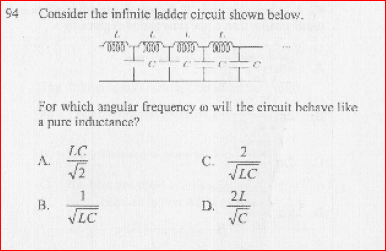# Infinite Ladder Circuit (Not with Resistors)

• Engineering
• vivekrai
In summary: C as my intuition told me in the first place.In summary, the conversation focused on solving a circuit for resistances with DC supply, using the total impedance of the circuit as Z and replacing one block with an inductor with impedance Z. However, the conversation became stuck when trying to add another inductor and capacitor pair to the circuit. Suggestions were made to use the ladder method and set up an equation to find Z. Finally, it was determined that the answer was 1/ωC, as originally suggested by intuition.

## Homework Statement## Homework Equations

Capacitive Reactance Xc = 1/ωC ; Inductive Reactance XL = ωL ; Some Phasor Algebra etc.,

## The Attempt at a Solution

Well, Basically like we solve the circuit for resistances with DC Supply, I tried to assume the total impedance of the Circuit as Z. Then leaving one block, replace all by an Inductor with impedance Z.

This is where I'm getting stuck!

Bump! Mentors, If some one is not able to solve in this sub section kindly move it to the appropriate section where some people will answer this.

I have moved this to Engineering, Comp Sci, & Technology.

vivekrai said:

## The Attempt at a Solution

Well, Basically like we solve the circuit for resistances with DC Supply, I tried to assume the total impedance of the Circuit as Z.
Yes, that is how I have solved this problem.
Then leaving one block, replace all by an Inductor with impedance Z.

This is where I'm getting stuck!
I don't really understand what you did in this last part. I would focus on figuring out just what Z is first. To do that, realize that the impedance is still going to be Z if you add another inductor + capacitor pair to the left side of the circuit. So you can set up an equation:
Z = Impedance of: (inductor) in series with (parallel combination of capacitor and Z)​
Use the actual impedance expressions for the inductor and capacitor, and solve the equation for Z. The algebra gets a little tedious, but after you get an expression for Z, you can then look at the expression and figure out how to make it consistent with a pure inductance.

Look at the dimensions: which version can be angular frequency at all?

An LC circuit without resistors is singular at its resonant frequencies: the impedance can be zero or infinite. Try to build the ladder step by step with ω=1/√(LC). What do you get? Can you apply the "ladder method" ?

If you apply the "ladder method" what do you get for Z? Does the angular frequency C give a meaningful solution?

ehild

Last edited:
I think that Redbelly has outlined a valid method, and it seems that it's essentially what vivekrai said he'd tried. But without vivekrai supplying more of his effort I don't see how we can be sure, or how we can do much to help.

I will give one suggestion though. Let s = jω then the impedances of the inductor and capacitors are given by sL and 1/(sC). Use Redbelly's suggestion and find Z such that
$$Z = sL + \frac{Z\,\frac{1}{s C}}{Z + \frac{1}{s C}}$$
The algebra won't be too bad, and you'll end up with a quadratic in Z. From the roots of the equation (quadratic formula) you should be able to spot what s (and hence ω) needs to be in order to leave just an "sL" type term.

Thanks to everyone for taking interest in solving it, But since its too late here in India now (IST), I'' solve it and tell you people by tomorrow.

Yes, Got it thanks. The answer turned out to be (C)